## Interpolating Gridded Data

### Gridded Data Representation

#### Grid Representation

Converting Between Grid Formats.  To convert a 2-D grid output from `meshgrid` to the `ndgrid` format, transpose the coordinate matrices:

```[X_meshgrid,Y_meshgrid] = meshgrid(1:3, 1:5); [X1_ndgrid,X2_ndgrid] = ndgrid(1:3,1:5); isequal(X_meshgrid',X1_ndgrid) ans = 1 isequal(Y_meshgrid',X2_ndgrid) ans = 1```
You can also use the `permute` function.
```isequal(permute(X_meshgrid,[2 1]),X1_ndgrid) ans = 1```
To convert a 3-D `meshgrid` to `ndgrid`, transpose each page of the coordinate array. For a given array `my_array`, `permute(my_array, [2 1 3])` interchanges the rows and columns, and the net effect is the transposition of every page:
```[X_meshgrid,Y_meshgrid,Z_meshgrid] = meshgrid(1:3, 1:5, [1 2]); [X1_ndgrid,X2_ndgrid,X3_ndgrid] = ndgrid(1:3,1:5, [1 2]); isequal(permute(X_meshgrid,[2 1 3]),X1_ndgrid) ans = 1 isequal(permute(Y_meshgrid,[2 1 3]),X2_ndgrid) ans = 1 isequal(permute(Z_meshgrid,[2 1 3]),X3_ndgrid) ans = 1```

Grid Vectors.  The inputs that you pass to the grid functions are called grid vectors. The grid vectors implicitly define the grid. Consider two vectors, `x1gv = (1:3)` and `x2gv = (1:5)`. You can think of these vectors as a set of coordinates in the `x1` direction and a set of coordinates in the `x2` direction, like so:Each arrow points to a location. You can use these two vectors to define a set of grid points, where one set of coordinates is given by `x1gv` and the other set of coordinates is given by `x2gv`. When the grid vectors are replicated they form two coordinate arrays that make up the full grid: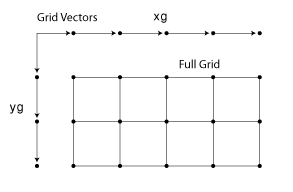Monotonic and Nonmonotonic Grids.  Your input grid vectors may be monotonic or nonmonotonic. Monotonic vectors contain values that either increase in that dimension or decrease in that dimension. Conversely, nonmonotonic vectors contain values that fluctuate. If the input grid vector is nonmonotonic, such as `[2 4 6 8 3 1]`, `ndgrid` outputs the following:

```[X1,X2] = ndgrid([2 4 6 3 1]) X1 = 2 2 2 2 2 4 4 4 4 4 6 6 6 6 6 3 3 3 3 3 1 1 1 1 1 X2 = 2 4 6 3 1 2 4 6 3 1 2 4 6 3 1 2 4 6 3 1 2 4 6 3 1```
Your grid vectors should be monotonic if you intend to pass the grid to other MATLAB® functions.

Uniform and Nonuniform Grids.  A uniform grid is one in which all neighboring points in a given dimension have equal spacing. For example, `[X1, X2] = ndgrid([1 3 5 9],[11 13 15])` is a uniform with a spacing of 2 units in each dimension.

It is not necessary for the spacing in a uniform grid to be equal in all dimensions. For example, ```[X1, X2] = ndgrid([1 2 3 4],[11 13 15])``` is considered uniform even though the spacing in `X1` and `X2` are different.

A grid whose spacing varies within any dimension is a nonuniform grid. For example, `[X1, X2] = ndgrid([1 5 6 9],[11 13 15])` creates a nonuniform grid because the spacing varies along the first dimension.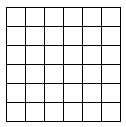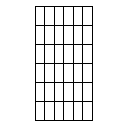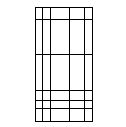Uniform Uniform Nonuniform

#### Types of Grid Representations

MATLAB allows you to represent a grid in one of three representations: full grid, compact grid, or default grid. The compact grid and default grid are used primarily for convenience and improved efficiency.

Full Grid.  A full grid is one in which the points are explicitly defined. The outputs of `ndgrid` and `meshgrid` define a full grid.

Compact Grid.  The explicit definition of every point in a grid is expensive in terms of memory. The compact grid representation is a way to dispense with the memory overhead of a full grid. The compact grid representation stores just the grid vectors instead of the full grid. (For information on how the `griddedInterpolant` class uses the compact grid representation, see Interpolation with the griddedInterpolant Class.)

Default Grid.  For many cases, when you are analyzing data, you care about both the distances between points in the grid and their value. For example, a data set might represent rainfall at specific points in a geographic area. In this case, you would care about the value at each grid point and the distance between a point and its neighbor. In other cases, you might care only about the value for a given point and not about the relative distances. For example, you could be working with input data from an MRI scan where the distance between the points is completely uniform. In this case, you would care about the values of the points, but you can be certain of a completely uniform grid. In this case, the default grid representation is useful. The default grid representation stores the value at a grid point explicitly and creates grid point coordinates implicitly.

#### Grid Approximation Techniques

In some cases, you may need to approximate a grid for your data. An approximate grid can be idealized by a standard MATLAB grid by choosing an appropriate set of grid vectors. For example, a grid can have points that lie along curved lines. A data set like this might occur if your data is longitude and latitude based: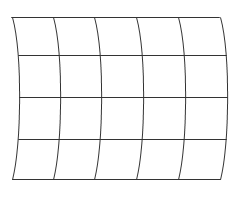In this case, although the input data cannot be gridded directly, you can approximate straight grid lines at appropriate intervals:You can also use the default grid.

Degenerate Grid.  A degenerate grid is a special case of grid where one or more dimensions of the grid are singletons. A singleton dimension can be internal, as in `7:7` in this example:

`[X1,X2,X3] = ndgrid(1:2:10,7:7,1:3:15);`
or a singleton dimension can be trailing:
`[X1,X2,X3] = ndgrid(1:2:10,1:3:15,7:7);`
You can create a degenerate grid if you are trying to take a slice of a larger data set. For example, you may want to analyze just a slice of a 3-D MRI scan. In this case, you will need a slice of data from a multidimensional grid, such as the dotted slice in the following figure:If you use indexing to extract the desired data, the resultant grid is degenerate in the `X3` dimension:

```[X1,X2,X3] = ndgrid(1:3); X1_slice = X1(:,:,2) X1_slice = 1 1 1 2 2 2 3 3 3 X2_slice = X2(:,:,2) X2_slice = 1 2 3 1 2 3 1 2 3 X3_slice = X3(:,:,2) X3_slice = 2 2 2 2 2 2 2 2 2```

The concept of data gridding is very important for understanding the ways in which MATLAB does grid-based interpolation.

### Grid-Based Interpolation

In grid-based interpolation, the data to be interpolated is represented by an ordered grid. For example, an arrangement of temperature measurements over a rectangular flat surface at 1-cm intervals from top-to-bottom vertically and left-to-right horizontally is considered 2-D gridded data. Grid-based interpolation provides an efficient way to approximate the temperature at any location between the grid points.

#### Benefits of Using Grid-Based Interpolation

Grid-based interpolation provides significant savings in computational overhead because the gridded structure allows MATLAB to locate a query point and its adjacent neighbors very quickly. To understand how this works, consider the following points on a 1-D grid:The lines connecting adjacent points represent the cells of the grid. The first cell occurs between `x = 1` and `x = 3`, the second occurs between `x = 3` and `x = 5`, and so on. Each number represents a coordinate in the grid. If you want to query the grid at `x = 6`, you would have to use interpolation because 6 is not explicitly defined in the grid. Since this grid has a uniform spacing of 2, you can narrow down the location of the query point with a single integer division (`6/2 = 3`). This tells you that the point is in the 3rd cell of the grid. Locating a cell in a 2-D grid involves performing this operation once in each dimension. This operation is called a fast lookup, and MATLAB uses this technique only when the data is arranged in a uniform grid.

This fast lookup efficiently locates the cell containing a query point `Xq`. A binary search proceeds as follows:

1. Locate the center grid point.

2. Compare `Xq` to the point at the center of the grid.

3. If `Xq` is less than the point found at the center, eliminate all of the grid points greater than central point from the search. Similarly, if `Xq` is greater than the one found at the center, we eliminate all of the grid points that are less than the central point. Note that by doing this, we have reduced the number of points we must search by half.

4. Find the center of the remaining grid points and repeat from Step 2 until you are left with one grid point on either side of your query. These two points mark the boundary of the cell that contains `Xq`.To illustrate the power of binary searches, consider the following example. Before the advent of electronic credit card authorizations, the only protection merchants had against fraudulent credit card purchases was to compare the account number on each customer's credit card against a list of "bad" account numbers. These lists were bound booklets with tens of thousands of card numbers arranged in ascending order. How many comparisons would be required to search through a list of 10,000 account numbers for one sale? It turns out that for any list of `n` ordered items, the maximum number of comparisons will be no more than the number of times you can divide the list in half, or `log2(n)`. Therefore, the credit card search will take no more than `log2(10e3)` or about 13 comparisons. That's a pretty impressive if you consider how many comparisons it would take to perform a sequential search.

By contrast, consider a problem with a scattered data set.

```x = rand(20,1); y = rand(20,1); scatter(x,y)```To find the points in close proximity to a query point would require many more operations. If your data can be approximated as a grid, grid-based interpolation will provide substantial savings in computation and memory usage.

If your data is scattered, you can use the tools detailed in Interpolating Scattered Data.

#### Interpolation versus Fit

The interpolation methods available in MATLAB create interpolating functions that pass though the sample data points. If you were to query the interpolation function at a sample location, you would get back the value at that sample data point. By contrast, curve and surface fitting algorithms do not necessarily pass through the sample data points.

#### Interpolation Methods

Grid-based interpolation offers several different methods for interpolation. When choosing an interpolation method, keep in mind that some require more memory or longer computation time than others. However, you may need to trade off these resources to achieve the desired smoothness in the result. The following table provides an overview of the benefits, trade-offs, and requirements for each method.

MethodDescriptionContinuityMemory Usage and PerformanceRequirements
Nearest NeighborThe interpolated value at a query point is the value at the nearest sample grid point. Discontinuous
• Modest memory requirements

• Fastest computation time

• Requires 2 grid points in each dimension.

Next NeighborThe interpolated value at a query point is the value at the next sample grid point.Discontinuous

Same memory requirements and computation time as nearest neighbor.

• Available for 1D interpolation only.

• Requires at least 2 grid points.

Previous NeighborThe interpolated value at a query point is the value at the previous sample grid point.Discontinuous

Same memory requirements and computation time as nearest neighbor.

• Available for 1D interpolation only.

• Requires at least 2 grid points.

LinearThe interpolated value at a query point is based on linear interpolation of the values at neighboring grid points in each respective dimension. C0
• Requires more memory than nearest neighbor.

• Requires more computation time than nearest neighbor.

• Requires at least 2 grid points in each dimension.

PchipThe interpolated value at a query point is based on a shape-preserving piece-wise cubic interpolation of the values at neighboring grid points.C1
• Requires more memory than linear.

• Requires more computation time than linear.

• Available for 1D interpolation only.

• Requires at least 4 grid points.

CubicThe interpolated value at a query point is based on cubic interpolation of the values at neighboring grid points in each respective dimension.C1
• Requires more memory than linear.

• Requires more computation time than linear.

• Grid must have uniform spacing, though the spacing in each dimension does not have to be the same.

• Requires at least 4 points in each dimension.

Modified AkimaThe interpolated value at a query point is based on a piecewise function of polynomials with degree at most three evaluated using the values of neighboring grid points in each respective dimension. The Akima formula is modified to avoid overshoots.C1
• Similar memory requirements as spline.

• Requires more computation time than cubic, but typically less than spline.

• Requires at least 2 grid points in each dimension

SplineThe interpolated value at a query point is based on a cubic interpolation of the values at neighboring grid points in each respective dimension.C2
• Requires more memory than cubic.

• Requires more computation time than cubic.

• Requires 4 points in each dimension.

This figure has some visual comparisons of the different interpolation methods for 1-D data.

Comparison of Interpolation MethodsMATLAB provides support for grid-based interpolation in several ways:

• The `interp` family of functions: `interp1`, `interp2`, `interp3`, and `interpn`.

• The `griddedInterpolant` class.

Both the `interp` family of functions and `griddedInterpolant` support N-D grid-based interpolation. However, there are memory and performance benefits to using the `griddedInterpolant` class over the `interp` functions. Moreover, the `griddedInterpolant` class provides a single consistent interface for working with gridded data in any number of dimensions.

### Interpolation with the interp Family of Functions

#### The interp1 Function

This example shows how the `interp1` function can be used to interpolate a set of sample values using the `'pchip'` method.

The function `interp1` performs one-dimensional interpolation. Its most general form is:

`Vq = interp1(X,V,Xq,method)`

where `X` is a vector of coordinates and `V` is a vector containing the values at those coordinates. `Xq` is a vector containing the query points at which to interpolate, and `method` optionally specifies any of four interpolation methods: `'nearest'`, `'linear'`, `'pchip'`, or `'spline'`.

Create a set of 1-D grid points `X` and corresponding sample values `V`.

```X = [1 2 3 4 5]; V = [12 16 31 10 6];```

Interpolate over finer intervals with 0.1 spacing.

```Xq = (1:0.1:5); Vq = interp1(X,V,Xq,'pchip');```

Plot the samples and interpolated values.

```plot(X,V,'o'); hold on plot(Xq,Vq,'-'); legend('samples','pchip'); hold off```#### 1-D Extrapolation With interp1

This example shows how to use the `'extrap'` option to interpolate beyond the domain of your sample points.

Define the sample points and values.

```X = [1 2 3 4 5]; V = [12 16 31 10 6];```

Specify the query points, `Xq`, that extend beyond the domain of `X`.

```Xq = (0:0.1:6); Vq = interp1(X,V,Xq,'pchip','extrap');```

Plot the results.

```figure plot(X,V,'o'); hold on plot(Xq,Vq,'-'); legend('samples','pchip'); hold off```In an alternative approach, you can introduce additional points to gain more control over the behavior in the extrapolated regions. For example, you can constrain the curve to remain flat in the extrapolated region by extending the domain with repeated values.

```X = [0 1 2 3 4 5 6]; V = [12 12 16 31 10 6 6];```

Specify the query points, `Xq`, that extend further beyond the domain of `X`.

`Xq = (-1:0.1:7);`

Interpolate using `'pchip'`. You can omit the `'extrap'` option because it is the default with the `'pchip'`, `'makima'`, and `'spline'` methods.

`Vq = interp1(X,V,Xq,'pchip');`

Plot the results.

```figure plot(X,V,'o'); hold on plot(Xq,Vq,'-'); legend('samples','pchip'); hold off```#### The interp2 Function

This example shows how the `interp2` function can be used to interpolate the coarsely sampled `peaks` function over a finer grid.

The `interp2` and `interp3` functions perform two and three-dimensional interpolation respectively, and they interpolate grids in the `meshgrid` format. The calling syntax for `interp2` has the general form:

`Vq = interp2(X,Y,V,Xq,Yq,method)`

where `X` and `Y` are arrays of coordinates that define a grid in `meshgrid` format, and `V` is an array containing the values at the grid points. `Xq` and `Yq` are arrays containing the coordinates of the query points at which to interpolate. `method` optionally specifies any of four interpolation methods: `'nearest'`, `'linear'`, `'cubic'`, or `'spline'`.

The grid points that comprise `X` and `Y` must be monotonically increasing and should conform to the `meshgrid` format.

Create a coarse grid and corresponding sample values.

```[X,Y] = meshgrid(-3:1:3); V = peaks(X,Y);```

Plot the sample values.

```surf(X,Y,V) title('Sample Grid');```Generate a finer grid for interpolation.

`[Xq,Yq] = meshgrid(-3:0.25:3);`

Use `interp2` to interpolate at the query points.

`Vq = interp2(X,Y,V,Xq,Yq,'linear');`

Plot the results.

```surf(Xq,Yq,Vq); title('Refined Grid');```#### The interp3 Function

This example shows how to use `interp3` to interpolate a 3-D function at a single query point and compare it to the value generated by an analytic expression.

`interp3` works in the same way as `interp2` except that it takes two additional arguments: one for the third dimension in the sample grid and the other for the third dimension in the query points,

`Vq = interp3(X,Y,Z,V,Xq,Yq,Zq,method)`.

As is the case with `interp2`, the grid points you supply to `interp3` must be monotonically increasing and should conform to the `meshgrid` format.

Define a function that generates values for X, Y, and Z input.

`generatedvalues = @(X,Y,Z)(X.^2 + Y.^3 + Z.^4);`

Create the sample data.

```[X,Y,Z] = meshgrid((-5:.25:5)); V = generatedvalues(X,Y,Z);```

Interpolate at a specific query point.

`Vq = interp3(X,Y,Z,V,2.35,1.76,0.23,'cubic')`
```Vq = 10.9765 ```

Compare `Vq` to the value generated by the analytic expression.

`V_actual = generatedvalues(2.35,1.76,0.23)`
```V_actual = 10.9771 ```

#### The interpn Function

This example shows how the `interpn` function can be used to interpolate a coarsely sampled function over a finer grid using the `'cubic'` method.

The function `interpn` performs n-dimensional interpolation on grids that are in the `ndgrid` format. Its most general form is:

`Vq = interpn(X1,X2,X3,...Xn,V,Y1,Y2,Y3,...,Yn,method)`

where `X1,X2,X3,...,Xn` are arrays of coordinates that define a grid in `ndgrid` format, and `V` is an array containing the values at the grid points. `Y1,Y2,Y3,...,Yn` are arrays containing the coordinates of the query points at which to interpolate. `method` optionally specifies any of four interpolation methods: `'nearest'`, `'linear'`, `'cubic'`, or `'spline'`.

The grid points that comprise `X1,X2,X3,...Xn` must be monotonically increasing and should conform to the `ndgrid` format.

Create a set of grid points and corresponding sample values.

```[X1,X2] = ndgrid((-5:1:5)); R = sqrt(X1.^2 + X2.^2)+ eps; V = sin(R)./(R);```

Plot the sample values.

```mesh(X1,X2,V) title('Sample Grid');```Create a finer grid for interpolation.

`[Y1,Y2] = ndgrid((-5:.5:5));`

Interpolate over the finer grid and plot the results.

```Vq = interpn(X1,X2,V,Y1,Y2,'cubic'); mesh(Y1,Y2,Vq) title('Refined Grid');````interpn` has an alternate syntax: `Vq = interpn(V,ntimes,method)` that allows you to interpolate over a grid that is an integer number of times finer than the sample grid. In the previous code, `Y1` and `Y2` queried the interpolant over a grid that contained one extra point between each of the samples. The following code demonstrates how you can achieve the same result with `ntimes=1`.

Interpolate over a finer grid using `ntimes=1`.

`Vq = interpn(V,1,'cubic');`

Plot the result.

```mesh(Vq) title('Refined Grid with NTIMES');```Notice that the plot is scaled differently than in the previous example. This is because we called `mesh` and passed the values only. The `mesh` function used a default grid based on the dimensions of `Vq`. The output values are the same in both cases.

You can also supply a nonuniform grid of query points. This can be useful if you are interested in querying the interpolant at a higher resolution in one region of the grid. The following code shows how this can be done.

Interpolate over a biased grid.

```[Y1, Y2] = ndgrid([-5 -4 -3 -2.5 -2 -1.5 -1.25 -1 -0.75 -0.5 -0.20 0]); Vq = interpn(X1,X2,V,Y1,Y2,'cubic');```

Plot the result.

```mesh(Y1,Y2,Vq); title('Biased Grid');```### Interpolation with the griddedInterpolant Class

Like the `interpn` function, the `griddedInterpolant` class provides a single interface for grid-based interpolation in n dimensions. However `griddedInterpolant` offers the following additional benefits:

• It offers substantial performance improvements for repeated queries to the interpolant.

• It offers additional performance improvements and savings in memory consumption because it stores the sample points as a compact grid.

`griddedInterpolant` accepts sample data that conforms to the `ndgrid` format. If you wish to create a `griddedInterpolant` with `meshgrid` data, you will need to convert the data to the `ndgrid` format. See Converting meshgrid Data to the ndgrid Format for a demonstration of how to convert 2-D and 3-D `meshgrids`.

The `griddedInterpolant` class supports the interpolation methods that are also supported by `interpn`: `nearest`, `linear`, `pchip`, `cubic`, `makima`, and `spline`. However `griddedInterpolant` offers greater performance with less overhead.

#### Constructing the Interpolant

An interpolant is a function that performs interpolation. You create the interpolant by calling the `griddedInterpolant` constructor and passing the sample data: the grid and corresponding sample values. You can also specify the interpolation method if you wish to override the default “linear” method. The calling syntax has the following forms:

• For 1-D interpolation, you can pass `x`, a set of points, and `v`, a vector of the same length containing the corresponding values.

`F = griddedInterpolant(x,v)`

• For higher dimensions, you can supply a full grid. `X1,X2,...,Xn` specify the grid as a set of n n-D arrays. These arrays conform to the `ndgrid` format and are the same size as the sample array `V`.

` F = griddedInterpolant(X1,X2,...,Xn,V)`

• If you know that the distances between adjacent sample points are uniform, you can let `griddedInterpolant` create a default grid by passing only the sample points `V`.

` F = griddedInterpolant(V)`

• You can also specify the coordinates of your sample data as a compact grid. The compact grid is represented by a set of vectors. These vectors are then packaged into a cell array by enclosing them in curly braces; for example,`{x1g,x2g,...,xng}` where vectors `x1g,x2g,...,xng` define the grid coordinates in each dimension.

` F = griddedInterpolant({x1g,x2g,...,xng},V)`

• You can also specify the interpolation method as a final input argument with any of the calling syntaxes. This example specifies nearest neighbor interpolation.

` F = griddedInterpolant({x1g,x2g,x3g},V,'nearest')`

#### Querying the Interpolant

The `griddedInterpolant`, `F`, is evaluated in the same way as you would call a function. Your query points may be scattered or gridded, and you can pass them to `F` in any of the following ways:

• You can specify an m-by-n matrix `Xq`, which contains m scattered points in n dimensions. The interpolated values `Vq` are returned as an m-by-1 vector.

` Vq = F(Xq)`

• You can also specify the query points as a series of n column vectors `x1q,x2q,...,xnq` of length m. These vectors represent m points in n dimensions. The interpolated values `Vq` are returned as an m-by-1 vector.

` Vq = F(x1q,x2q,...,xnq)`

• You can specify the query points as a series of n n-dimensional arrays representing a full grid. The arrays `X1q,X2q,...,Xnq` are all the same size and conform to the `ndgrid` format. The interpolated values `Vq` will also be the same size.

`Vq = F(X1q,X2q,...,Xnq)`

• You can also specify the query points as a compact grid. `x1gq,x2gq,...,xngq` are vectors that define the grid points in each dimension.

`Vq = F({x1gq,x2gq,...,xngq})`

For example, in 2-D:

`Vq = F({(0:0.2:10),(-5:0.5:5)});`

#### Converting meshgrid Data to the ndgrid Format

The `griddedInterpolant` class accepts `ndgrid` formatted sample data. If you want to create a `griddedInterpolant` with `meshgrid` data, you should convert it to the `ndgrid` format first.

The following example outlines the steps for converting 2-D `meshgrid` data to the `ndgrid` format. We begin by creating the `meshgrid` and corresponding sample values:

```[X,Y] = meshgrid(-2:.1:2,-1:.1:1); V=0.75*Y.^3-3*Y-2*X.^2;```

To convert `X`, `Y`, and `V` to `ndgrid` format, follow these steps:

1. Transpose each array in the grid as well as the sample data.

```X=X'; Y=Y'; V=V';```
2. Now create the interpolant.

`F = griddedInterpolant(X,Y,V);`

To convert a 3-D `meshgrid`, use the `permute` function. Again, start by creating the `meshgrid` and corresponding sample values:

```[X,Y,Z] = meshgrid(-5:5,-3:3,-10:10); V = X.^3 + Y.^2 + Z;```

To convert `X`, `Y`, `Z`, and `V` to `ndgrid` format, follow these steps:

1. Use the `permute` function to interchange the rows and columns of each array. The net effect will be the transpose of every page.

```P = [2 1 3]; X = permute(X,P); Y = permute(Y,P); Z = permute(Z,P); V = permute(V,P);```

2. Now create the interpolant.

`F = griddedInterpolant(X,Y,Z,V);`

#### griddedInterpolant in One Dimension

This example shows how to create and plot a 1-D interpolant using `griddedInterpolant` with a cubic interpolation method.

Create a coarse grid and sample values.

```X = [1 2 3 4 5]; V = [12 6 15 9 6];```

Construct the `griddedInterpolant` using a cubic interpolation method.

`F = griddedInterpolant(X,V,'cubic')`
```F = griddedInterpolant with properties: GridVectors: {[1 2 3 4 5]} Values: [12 6 15 9 6] Method: 'cubic' ExtrapolationMethod: 'cubic' ```

The `GridVectors` property contains the compact grid specifying the coordinates of the sample values `V`. The `Method` property specifies the interpolation method. Notice that we specified `'cubic'` when we created `F`. If you omit the `Method` argument, the default interpolation method, `linear`, will be assigned to `F`.

You can access any of the properties of `F` in the same way you would access the fields in a `struct`.

```F.GridVectors; % Displays the grid vectors as a cell array F.Values; % Displays the sample values F.Method; % Displays the interpolation method```

Interpolate over finer intervals with 0.1 spacing.

```Xq = (1:0.1:5); Vq = F(Xq);```

Plot the result.

```plot(X,V,'o'); hold on plot(Xq,Vq,'-'); legend('samples','Cubic Interpolation');```#### griddedInterpolant in Two Dimensions

This example shows how to create and plot a 2-D interpolant using `griddedInterpolant`.

In two dimensions and higher, you can specify the sample coordinates as an `ndgrid`, a compact grid, or a default grid. In this example, we'll supply an `ndgrid`.

Create a coarse grid and sample values.

```[X,Y] = ndgrid(-1:.3:1,-2:.3:2); V = 0.75*Y.^3 - 3*Y - 2*X.^2;```

Construct the `griddedInterpolant`.

`F = griddedInterpolant(X,Y,V,'spline');`

Interpolate over finer intervals with 0.1 spacing.

```[Xq,Yq] = ndgrid(-1:.1:1,-2:.1:2); Vq = F(Xq,Yq);```

Plot the result.

```figure() surf(X,Y,V); view(65,60) title('Sample Data');``````figure() surf(Xq,Yq,Vq); view(65,60) title('Refined with Spline');```#### griddedInterpolant in Three Dimensions

This example shows how to create a 3-D interpolant and evaluate over a slice plane so you can plot the values on that plane.

Create a full grid and sample values.

```[X,Y,Z] = ndgrid((-5:2:5)); V = X.^3 + Y.^2 + Z.^2;```

Construct the `griddedInterpolant`.

`F = griddedInterpolant(X,Y,Z,V,'cubic');`

Since we already created the full grid to generate our sample values, we had nothing to lose in passing it to `griddedInterpolant`. In practice however, it's common to load the sample data into MATLAB from disk. The compact grid can very beneficial in such cases because it allows you to specify the entire grid in a form that is much more economical in terms of memory. If we had loaded `V` into MATLAB instead of calculating it from a full grid, we could have created a compact grid to conserve memory in our workspace. For example,

```gv = {(-5:2:5),(-5:2:5),(-5:2:5)}; F = griddedInterpolant(gv,V,'cubic');```

Now interpolate over a plane at `Z = 2` with 0.25 spacing.

```[Xq,Yq,Zq] = ndgrid((-5:.25:5),(-5:.25:5),2:2); Vq = F(Xq,Yq,Zq);```

Plot the result.

```surf(Xq,Yq,Vq); title('Refined with Linear Interpolation');```#### griddedInterpolant in Four Dimensions

This example creates a 4-D interpolant and evaluates it at a single point.

1. Create a coarse grid and sample values.

```[X1,X2,X3,X4] = ndgrid((-5:2:5)); V = X1.^3 + X2.^2 + X3.^2 +X4;```

2. Construct the `griddedInterpolant`.

`F = griddedInterpolant(X1,X2,X3,X4,V,'linear');`

3. Query the `griddedInterpolant` at a single point.

`Vq = F([-3.2 2.1 4.7 -1.3])`

MATLAB outputs the following:

```ans = -10.1000```

.

#### Other Ways of Working with griddedInterpolant

Depending on the arrangement of your query points, you may prefer one evaluation syntax over the others. For example, create the following interpolant:

```[X,Y] = ndgrid(-1:.25:1,-2:.25:2); V = 0.75*Y.^3-3*Y-2*X.^2; F = griddedInterpolant(X,Y,V);```

Query `F` using a full grid to give the values at the grid points:

```[Xq,Yq] = ndgrid(-1:.1:0,-2:.1:0); Vq = F(Xq,Yq);```

You can also interpolate over the same grid using the compact grid format:

```gvq = {-1:.1:0,-2:.1:0}; Vq = F(gvq);```

Or you can query a single point:

```Vq = F(.315,.738) ```

which returns:

```Vq = -2.1308 ```

or a random set of scattered points:

```rng('default') Vq = F(rand(3,2))```

which returns:

```Vq = -3.4919 -3.3557 -0.3515```

You can also examine the `Values` in `F`:

``` F.Values(1,3) ```

which returns:

``` ans = -0.0313```

Or you can replace the `Values` array:

`F.Values = 2*V;`

You can edit the properties in `F` on-the-fly. For example, you can replace the interpolation method as follows:

``` F.Method = 'cubic'; ```

You can also replace the `GridVectors` in `F`. First, examine `GridVectors`:

```gv = F.GridVectors; gv{1}```
`gv` is a cell array, and `gv{1}` displays the first grid vector:
```ans = -1.0000 -0.7500 -0.5000 -0.2500 0 0.2500 0.5000 0.7500 1.0000```

Now replace the `GridVectors` in `F` by creating a new cell array `new_gv`:

```new_gv = {(0:0.3:1),(0:0.3:1)}; F.GridVectors = new_gv;```